## Exploring the local dynamics of : Larter-Breakspear model

In this tutorial we will explore the dynamics of a modified verion of the Larter model (Larter et al., 1999) as presented in the work of (Breakspear et al., 2003).

For the uncoupled model, ie analyzing only the dynamics of a single neural mass model, we will use a simple version in python using scipy odeint integration scheme; the same equations have been implemented in TVB although the public repo hasn't been updated on github yet to see those changes.

First, it would be nice to reproduce the figures presented in (Breakspear et al., 2003) to get an idea of the parameter settings that could be used in the coupled case.

## The Model

This model is a 3 dimensional model. It consists of three state variables; two representing the membrane potential of one excitatory and one inhibitory population; the third state variable is the number of open potassium channels.

• $V$: The average membrane potential of the excitatory (pyramidal neurons);
• $Z$: The average membrane potential of the inhibitory (interneurons); and
• $W$: The average number of open potassium ion channels.

The evolution equations are:

$\dfrac{dV}{dt} = - (g_{Ca} + (1 - C) \, r_{NMDA} \, a_{ee} \, Q_V \\ + C \, r_{NMDA} \, a_{ee} \, \langle Q_V\rangle) \, m_{Ca} \, (V - VCa)\\ - g_K \, W \, (V - VK) - g_L \, (V - VL) - (g_{Na} \, m_{Na} + (1 - C) \, a_{ee} \, Q_V \\ + C \, a_{ee} \, \langle Q_V\rangle) \,(V - VNa) + a_{ei} \, Z \, Q_Z + a_{ne} \, I$

NOTE: However in this equation the term $aei \, Z \, QZ$ should be preceded by a $-$ not a $+$. Having an addition term makes the system numerically unstable and the variables grow to infinity. The 'corrected' version is used in this tutorial. I think that the code used in (Breakspear et al., 2003; Honey et al., 2007 and Honey et al. 2009) had the sign properly set; it'd be nice to have the code to verify this.

$\dfrac{dW}{dt} = \phi \, \dfrac{m_K - W}{\tau_{K}}$

$\dfrac{dZ}{dt} = b \left[ a_{ni}\, I + a_{ei}\,V\,Q_V\right]$

The relationship between membrane voltage and percentage of open channels is given by an activation function:

$m_{Ca} = 0.5 \, (1 + \tanh((V - TCa) / \delta_{Ca}))$

$m_{Na} = 0.5 \, (1 + \tanh((V - TNa) / \delta_{Na}))$

$m_{K} = 0.5 \, (1 + \tanh((V - TK ) / \delta_K))$

Finally the voltage-to-firing rate neural activation function:

$Q_V = 0.5 * Q_{V_{max}} \, (1 + tanh((V - VT) / \delta_{V}))$

$Q_Z = 0.5 * Q_{Z_{max}} \, (1 + tanh((Z - ZT) / \delta_Z))$

In :
# Some useful imports
import numpy
from scipy.integrate import odeint

In :
#"""
#Parameters from Table 1 (Breakspear et al., 2003)
#"""

# Conductances
gCa = 1.1
gK  = 2.0
gL  = 0.5
gNa = 6.7
rNMDA = 0.25
# Scaling factors
phi = 0.7
tau_K = 1.0
b   = 0.1
# Ion threshold values
TK  =  0.0
TCa = -0.01
TNa =  0.3
d_K =  0.3
d_Na = 0.15
d_Ca = 0.15
# Nernst potentials
VCa =  1.0
VK  = -0.7
VL  = -0.5
VNa =  0.53
# voltage to firing rate
VT  = 0.0
d_V = 0.5 # this parameter is varied throughout the text
ZT  = 0.0
d_Z = 0.7
QV_max = 1.0
QZ_max = 1.0
# Coupling terms
aei = 2.0
aie = 2.0
aee = 0.5
ane = 1.0
ani = 0.4
C   = 0.0
# External
Iext = 0.3

In :
# define time derivatives
def dfun(y, t):
"""
Time derivatives LarterBreakspear model...
"""

V = y
W = y
Z = y

# voltage-to-fraction of open channels
m_Ca = 0.5 * (1 + numpy.tanh((V - TCa) / d_Ca))
m_Na = 0.5 * (1 + numpy.tanh((V - TNa) / d_Na))
m_K  = 0.5 * (1 + numpy.tanh((V - TK ) / d_K))

# voltage-to-firing rate
QV = 0.5 * QV_max * (1 + numpy.tanh((V - VT) / d_V))
QZ = 0.5 * QZ_max * (1 + numpy.tanh((Z - ZT) / d_Z))

# NOTE: For all the following examples C=0.
#       The term C*rNMDA*aee*QV and C * aee * QV are only placeholders to follow equation .

dV = (- (gCa + (1.0 - C) * rNMDA * aee * QV + C * rNMDA * aee) * m_Ca * (V - VCa) - gK * W * (V - VK) -  gL * (V - VL) - \
(gNa * m_Na + (1.0 - C) * aee * QV + C * aee ) * (V - VNa) - aei * Z * QZ + ane * Iext)

dW = phi * (m_K - W) / tau_K
dZ = b * (ani * Iext + aei * V * QV)

return [dV, dW, dZ]

In :
# set some initial conditions
V0 = -0.12              # initial membrane potential
W0 = 0                  # initial potassium channels
Z0 = 0                  # initial memembrane potential
y0 = [V0, W0, Z0]       # initial condition vector

In :
# integration time as in Honey et al., 2007, 2009, Alstott et al., 2009
# time scale is to be interpreted as in milliseconds
t = numpy.arange(0.0, 2000, .2)

In :
# solve ODEs
soln_2 = odeint(dfun, y0, t)

In :
# imports to create pretty pictures
import matplotlib.pyplot as plt
from numpy import linspace
from mpl_toolkits.mplot3d import Axes3D

In :
# define some plotting functions

def trajectory_3d(sv):
# get integrated traces
V, W, Z = sv[:, 0], sv[:, 1], sv[:, 2]
fig = plt.figure()
ax.plot(V, W, Z, linewidth=2)
ax.set_xlabel('V')
ax.set_ylabel('W')
ax.set_zlabel('Z')

def time_series(sv, f=0.1):
"""
sv: integrated time series
f: fraction of the time series to display for the second subplot (zoom)
"""
# get integrated traces
V, W, Z = sv[:, 0], sv[:, 1], sv[:, 2]
# time-series
subplot(121);plot(t, V, linewidth=2)
t_end = int(t.shape * f)
subplot(122);plot(t[0:t_end], V[0:t_end], linewidth=4)



## Reproducing figures from (Breakspear et al., 2003)

### Figure 2: Fixed point attractor

With the parameters as per table 1 and $\delta_V < 0.55$ (here it's set to 0.5), the system should exhibit fixed point attractor dynamics. In the caption it says $VT=0.54$. However, if this value is used the system becomes unstable. Also, $d_V=0.54$ and default parameters, the trajectory looks like Fig. 5a). See NOTES below.

In :
trajectory_3d(soln_2)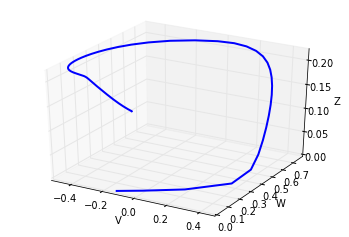In :
time_series(soln_2, f=0.02)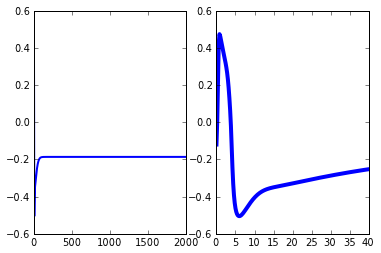Remarks: While we obtain the fixed point dynamics, the scale is not the same as the one presented in the original figure.

## Figure 3. Limit cycle

According to the text and figure caption parameters are as per table 1 except $VT$=0.56 and $\delta_V = 0.56$.

In :
# Change the corresponding parameters
VT  = 0.56
d_V = 0.56

In :
# solve ODEs for Figure 3
soln = odeint(dfun, y0, t)

In :
trajectory_3d(soln)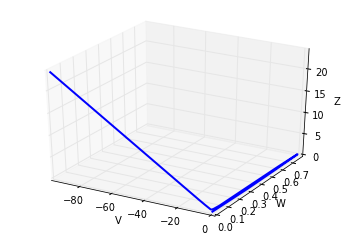Remarks: My impression is that in the text the parameter $\delta_V$ is specified. Then in the caption is said to be $V_T$. This poses a problem because setting both parameters according to the main text and captions does not lead to the figures presented in the article.

In :
# reset VT and simulate again
VT=0.0
soln_3 = odeint(dfun, y0, t)

In :
trajectory_3d(soln_3)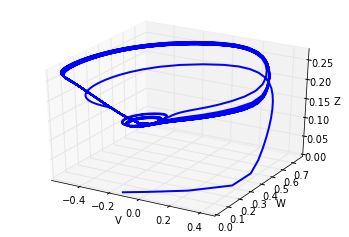Remarks This trajectory resembles more to Figure 5 a) where the system is said to exhibit a chaotic behaviour.

In :
time_series(soln_3, f=0.1)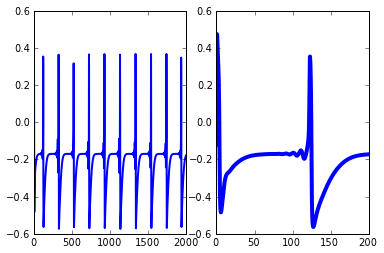## Figure 4. Period 9 limit cycle

The values given for this figure are $\delta_V=0.6$, $a_{ee}=0.5$, $a_{ie}=0.5$, $g_{Na}=0.0$, $I=0.165$. The rest of the parameters as per table 1.

In :
# Change the corresponding parameters
d_V = 0.6
aee = 0.5
aie = 0.5
gNa = 0.0
I   = 0.165
VT = 0.0

In :
# solve ODEs for Figure 4
soln_4 = odeint(dfun, y0, t)

In :
trajectory_3d(soln_4)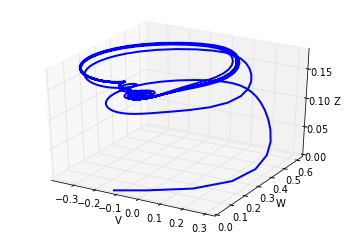Remarks This setting more or less corresponds to what we see in Figure 4 in the article. The time series are not exactly the same though.

In :
time_series(soln_4, f=0.1)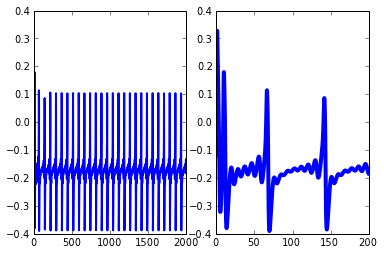## Figure 5. Chaotic behaviour

$\delta_v=0.65$. The rest of the parameters as per table 1. In the caption it says "for VT=0.65", however using this value will lead to an unstable behaviour.

In :
# Change the corresponding parameters
d_V = 0.65
aee = 0.4
aie = 2.0
gNa = 6.7
I   = 0.3

In :
# solve ODEs for Figure 5
soln_5 = odeint(dfun, y0, t)

In :
trajectory_3d(soln_5)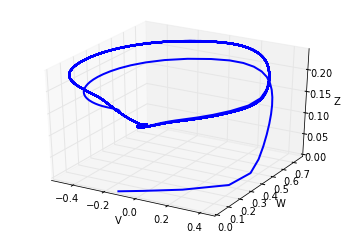Remarks This setting more or less corresponds to what we see in Figure 5 a) in the article.

In :
time_series(soln_5, f=0.05)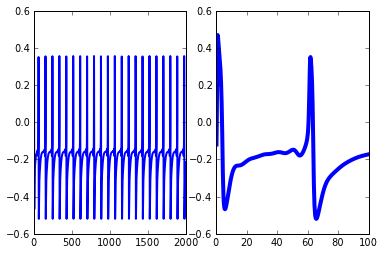### The limit cycle attractor

To obtain Figure 3, the parameters used were $\delta_V=0.6$, $a_{ee}=0.5$, $a_{ie}=0.5$, $g_{Na}=0.0$, $I=0.165$ and $a_{ni}=0.1$. The rest of the parameters were as per table 1.